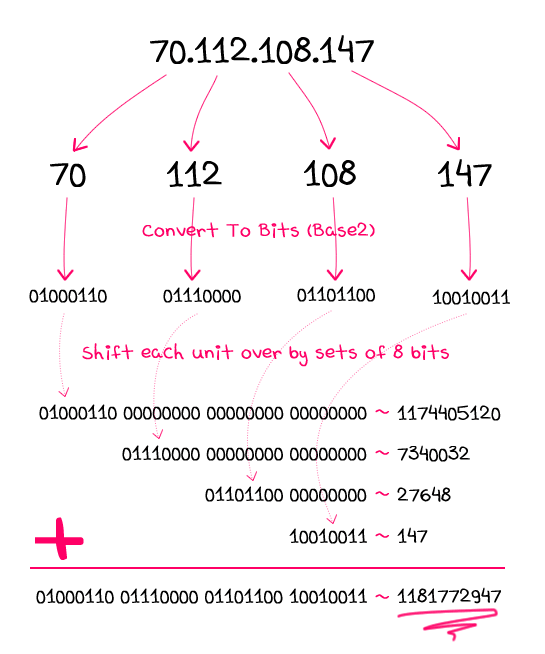My current Splunk deployment is ingesting custom McAfee EPO data through Splunk Enterprise Security (ES). We are developing many use cases around this data that require us to alert/output an IP address. Currently, the McAfee EPO provides an IP address in integer form (i.e. 2130706433) and not in string/readable form (i.e. 127.0.0.1). In order to make the IP address readable and valuable to the user, we need to convert the IP address from an integer to a string. Below, is an example of the conversion as well as the Splunk search-time conversion I used. I will calculate each octet in order to determine the final IP address.

Example – IP Integer to String

IP integer:      2130706433

The Conversion -

Calculating Octet 1:

```octet_1      =   ip_integer / (256 * 256 * 256)
127.00000006 =   2130706433 / 16777216
octet_1   =   ~127 (round down)

rest_of_ip =   ip_integer – (octet_1 * (256 * 256 * 256))
1 =   2130706433 – (127 * 16777216)```

Calculating Octet 2:

```octet_2    =   rest_of_ip / (256 * 256)
0.00001526 =   1 / 65536
octet_2   =   ~0 (round down)

new_rest_of_ip =   rest_of_ip – (octet_2 * (256 * 256 ))
1 =   1 – (0 * 65536)```

Calculating Octet 3:

```octet_3    =   new_rest_of_ip / 256
0.00390625 =   0 / 256
octet_3   =   ~0 (round down)```

Calculating Octet 4:

```octet_4   =   new_rest_of_ip – (octet_3 * 256)
1   =   1 - (0 * 256)
octet_4   =   1```

```IP     =     octet_1 . octet_2 . octect_3 . octet_4

IP     =     127.0.01```

Splunk Search – IP Integer to String

```index = mcafee_epo sourcetype = <sourcetype>

| eval oct_1 = floor(<ip_integer>/16777216)
| eval restOfIP = <ip_integer>-(oct_1*16777216)

| eval oct_2 = floor(restOfIP/65536)
| eval restOfIP = restOfIP-(oct_2*65536)

| eval oct_3 = floor(restOfIP/256)

| eval oct_4 = (restOfIP-(oct_3*256))

| eval src_ipv4 = oct_1.".".oct_2.".".oct_3.".".oct_4

| stats count by src_ipv4 <ip_integer> ...```

The above conversion has been extemely important in developing our McAfee EPO use cases. It has given us a better understanding of our data and environment. I hope it proves to be as valuable for you, as it has been for me.